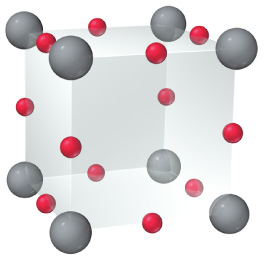# Problem: An oxide of uranium crystallizes with the following unit cell (uranium = gray; oxygen = red) What is the formula of the oxide?

###### FREE Expert Solution

We are asked to identify the formula of this oxide.

It is given that uranium = gray; oxygen = red.

First, let us count how many atoms are there for each element in the unit cell.

93% (87 ratings)###### Problem Details

An oxide of uranium crystallizes with the following unit cell (uranium = gray; oxygen = red) What is the formula of the oxide?